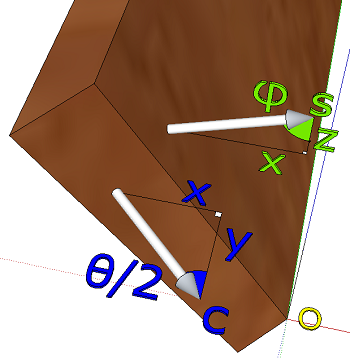# Derivation of Equations for Compound Angles in the N-sided Box

This page is for those of you who are interested in how I arrived at the equations used in the N-sided box calculator. The equations can be found in the javascript section of the calculator's html file.

I found the original equations at the now defunct Scarletta site, and I became curious about how they were derived. I could not find any clues anywhere so I set out to derive them myself.

## SetupThe assembled box is sitting with its inside bottom edge on the XY-plane, oriented such that one side is parallel with the Y-axis. That side's inside bottom corner is at the origin.

Two inputs define the angles of the box:

• φ is the angle the sides make with the XY-plane
• n is the number of sides in the box

From n we can calculate the angle θ which separates the n cutting planes in the XY-plane.

θ = 360° / n

## MiterConsider now the one piece that lays on the Y-axis. The triangle OPQ is on the inside surface of the board with sides h and y, and it includes the miter angle. The relationship between y and h is:

y / h = tan miter

The triangle OPQ projected on the XY-plane has angle θ/2 and sides x and y. The relationship between x and y is:

y / x = tan( θ/2 )

In the XZ-plane the distance OP is the height h of the board, which showed as x in the XY-plane. The relationship between h and x is:

x / h = cos φ

We solve for the miter angle by substituting from the three equations above:

miter = tan-1 y/h
= tan-1[ x tan( θ/2 ) / {x / cos( φ )} ]
= tan-1[ cos( φ ) tan( θ/2 ) ]

## BevelWe define a unit surface normal s for the inner surface of the Y-axis piece, pointing into the box. If this side were perpendicular to the bottom then its normal would have the xyz-components <1, 0, 0>, but since the side is tilted outward by an angle φ w.r.t. horizontal, its components are really this:

s = < sin φ, 0, cos φ >

The cutting plane that includes the Origin has a unit normal c with the following components:

c = < sin θ/2, -cos θ/2, 0 >

We can find the angle β between the side surface and the cutting plane, which is the same as the angle between their normals. We find β using the geometric definition of the dot product:

s·c = |s| |c| cos β
...where |s|=1 and |c|=1

Solving for β:

β = cos-1( s·c )
= cos-1[ < sin φ, 0, cos φ > · < sin θ/2, -cos θ/2, 0 > ]
= cos-1[ sin( φ ) sin( θ/2 ) ]

The bevel angle is the complement to β.

bevel = 90° - cos-1[ sin( φ ) sin( θ/2 ) ]
= sin-1[ sin( φ ) sin( θ/2 ) ]

Reference: The Calculus with Analytic Geometry, 6th Edition, Louis Leithold, ISBN 0-06-043930-0